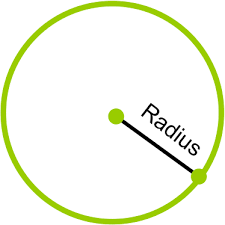Problems
337 - Circumference

Circumference

Time Limit: 1 sec

The Problem

Radius of a Circle, R is given. What will be the Circumference of the Circle?

Note : The Circumference of a Circle is 2 * pi * radius [pi = 3.1416]The Input

The input file contains a series of line. Each line contains a single integer Radius R (R <= 100). Input is terminated by EOF.

The Output

The output will show the Circumference of the Circle rounded to 2 decimal points in a separate line.

Sample Input

2

5

Sample Output

12.57

31.42

Problem Setter: Samia Safa Ahmed